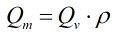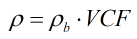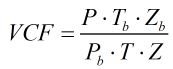# C248 – Gas Flow Rate Conversion from Qv

## Description

This calculates an observed volume flow rate of a gas to a mass, standard volume, normal volume or energy flow rate.

Kelton calculation reference C248

## Options

### Calculate for

• Observed volume
• Mass
• Standard volume
• Normal volume
• Energy

This option group is used to select which flow rate(s) are needed to be output.

### Method

• Density
• Volume correction factor

This option allows the user to select whether to calculate a volumetric type flow rate using the equivalent gas density or by using a volume correction factor.

### Calculate mass using

• Line density
• Standard density

### Volume correction factor

• User entered
• Calculated

This option allows the user to enter a volume correction factor or have it calculated using PTZ inputs.

### Calorific Value

• Volume based
• Mass based

This option allows the user to enter either a volume or mass based calorific value when calculating energy flow rate.

### Standard density

• User entered
• Input as relative density

This option allows the user to select whether to enter standard density or enter a relative density, which will then be used to calculate standard density.

## Calculation

### Mass Flow Rate

The mass flow rate is calculated by:If the density is calculated it is done by:If the volume correction factor is calculated it is done by:Where P = Pressure at line conditions Pb = Pressure at base conditions T = Temperature at line conditions Tb = Temperature at base conditions Z = Compressibility at line conditions Zb = Compressibility at base conditions ρ = Density at line conditions ρb = Density at base conditions Qv = Observed volume flow rate

### Standard Volume Flow Rate

The standard density is calculated by:

If the density method option is selected:If volume correction factor option is selected:### Energy Flow Rate

The energy flow rate is calculated by:

If volumetric calorific value is applied:If mass based calorific value is applied:Back to FLOCALC Calculations